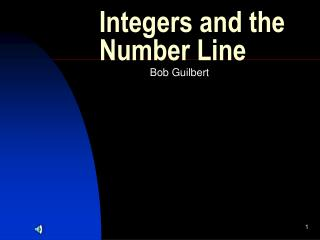DownloadDownload PresentationIntegers and the Number Line

Integers and the Number Line

Télécharger la présentationIntegers and the Number Line

- - - - - - - - - - - - - - - - - - - - - - - - - - - E N D - - - - - - - - - - - - - - - - - - - - - - - - - - -
Presentation Transcript

1. Integers and the Number Line Bob Guilbert

2. Constructing a Number Line • A number line is drawn by choosing a starting position, usually 0, and marking off equal distance from that point

3. Although only a portion of the number line is shown, the arrowheads indicate that the line and the set of numbers continue

4. The set of numbers used on the number line is called the set of integers • This set can be written {…, -3, -2, -1, 0, 1, 2, 3, …}

5. The number line is made up of 3 sets of numbers • Natural numbers 1, 2, 3, 4, … • Whole numbers 0, 1, 3, 4, … • Integers …, -2, -1, 0, 1, 2, …

6. We can use a Venn Diagram to represent thee 3 sets of numbers and their relationship to one another

7. You should not include the number 0 • You should not include numbers less than 0 (negative numbers) • You should not include the number 6 or any higher

8. Positive numbers move to the right • Negative numbers move to the left • + 3 + (-8) = -5

9. In Summary